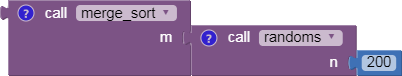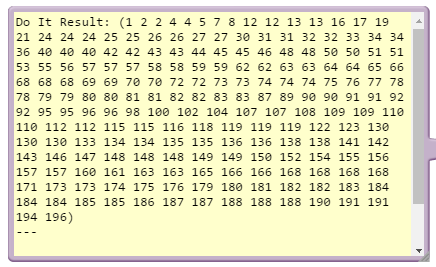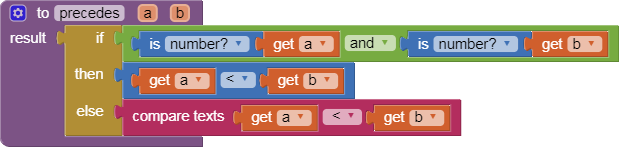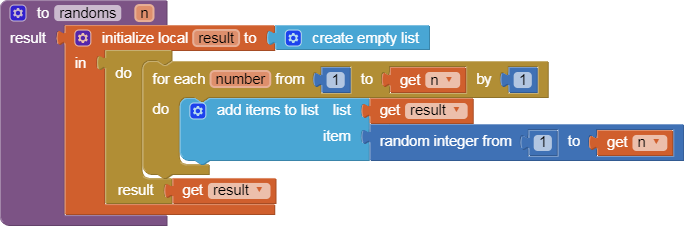# Merge Sort Block Procedures Library

This is a set of procedures you can use to sort lists and tables, using your own test criterion for greater/lesser comparison. It is based on the merge sort algorithm, which runs at n*log(n) speed. The procedure blocks should be draggable directly into your Blocks Editor workspace.

merge_sort.aia (4.9 KB)The logic:

function merge_sort(list m)
// Base case. A list of zero or one elements is sorted, by definition.
if length of m ≤ 1 then
return m

``````// Recursive case. First, divide the list into equal-sized sublists
// consisting of the first half and second half of the list.
var left := empty list
var right := empty list
for each x with index i in m do
if i ≤ (length of m)/2 then
else

// Recursively sort both sublists.
left := merge_sort(left)
right := merge_sort(right)

// Then merge the now-sorted sublists.
return merge(left, right)
``````

Merge function logic:

function merge(left, right)
var result := empty list

``````while left is not empty and right is not empty do
if first(left) ≤ first(right) then
append first(left) to result
left := rest(left)
else
append first(right) to result
right := rest(right)

// Either left or right may have elements left; consume them.
// (Only one of the following loops will actually be entered.)
while left is not empty do
append first(left) to result
left := rest(left)
while right is not empty do
append first(right) to result
right := rest(right)
return result``````
7 Likes

Thank you for this merge library!